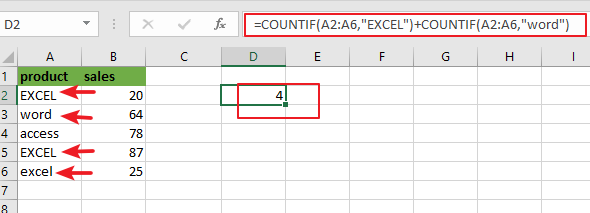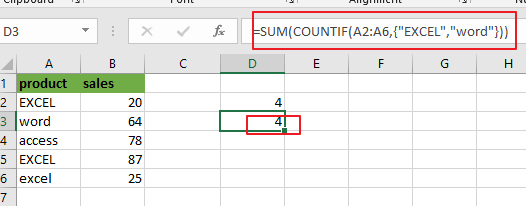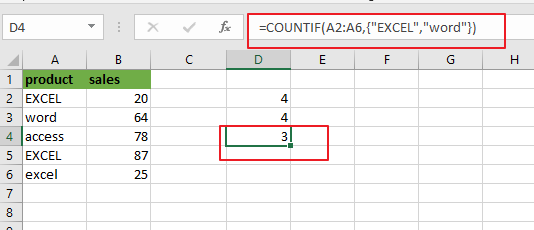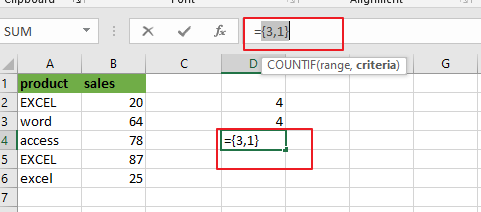# How to Count Cells equal to X or Y in Excel

This post will guide you how to count the number of cells equal to criteria X or Y in a given range cells using a formula in Excel 2013/2016.You can easily to count cells equal to a specific value through COUNTIF function. But if there is an easy way to count cells equal to either one value or another value in a selected range of cells in Excel.

## Count Cells equals to Criteria X or Y

Assuming that you want to count cells that contain value “EXCEL”or “word” defined in a selected range(A1:B6).In this case, you can use the COUNTIF function twice to count cells which are equal to X or Y.

Enter the following formula in a blank cell, and press Enter key:

=COUNTIF(A2:A6,”EXCEL”)+COUNTIF(A2:A6,”word”)Note: A2:A6 is the data range that you want to use. And both“`EXCEL`” and “word” are the text values that you want to count in range `A2:A6`. You need to change them as you need.

Now Let’s see how this formula works:

The COUNTIF function will count the number of cells in a given range that match supplied criteria. In the above formula, there are two COUNTIF function, and contain two criteria.

One criteria is “EXCEL”, another is “word”. The first COUNTIF function returns the number of count equal to “EXCEL”in A2:A6.

The second COUNTIF function returns the count of cells equal to criteria “word” in the range A2:A6. The formula adds both results returned by these two COUNTIF function and return a final result.

Note: you need to know that the COUNTIF function is not case sensitive. And if you want to count the number of cells in a range with case-sensitive, you need to use EXACT function.

You can also use another array formula to achieve the same result of counting cells equal to either “EXCEL” or “word” in A2:A6. Just using the following formula based on the SUM function and the COUNTIF function:

=SUM(COUNTIF(A2:A6,{“EXCEL”,”word”}))Since two criteria were provided in the COUNTIF function, it will return an array result that containing multiple result. You can press “`Fn`” + “`F9`” to display the array result. Like below:{3,1}

The SUM function will add this array results and return a final result of 4.

### Related Functions

• Excel COUNTIF function
The Excel COUNTIF function will count the number of cells in a range that meet a given criteria. This function can be used to count the different kinds of cells with number, date, text values, blank, non-blanks, or containing specific characters.etc.= COUNTIF (range, criteria)…
• Excel EXACT function
The Excel EXACT function compares if two text strings are the same and returns TRUE if they are the same, Or, it will return FALSE.The syntax of the EXACT function is as below:= EXACT (text1,text2)…
• Excel SUM function
The Excel SUM function will adds all numbers in a range of cells and returns the sum of these values. You can add individual values, cell references or ranges in excel.The syntax of the SUM function is as below:= SUM(number1,[number2],…)…